Next: Two-State System Up: Time-Dependent Perturbation Theory Previous: Introduction

# General Analysis

Suppose that at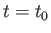the state of the system is represented by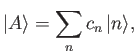(8.3)

where the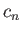are complex numbers. Thus, the initial state is some linear superposition of the unperturbed energy eigenstates. In the absence of the time-dependent perturbation, the time evolution of the system is given by(8.4)

(See Section 3.5.) Here,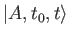is the state ket of the system at time, given that the state ket at the initial timeis. Now, the probability of finding the system in state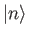at timeis(8.5)

Clearly, with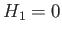, the probability of finding the system in stateat timeis exactly the same as the probability of finding the system in this state at the initial time,. However, with, we expect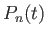to vary with time. Thus, we can write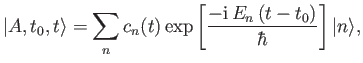(8.6)

where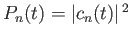. Here, we have carefully separated the fast phase oscillation of the eigenkets, which depends on the unperturbed Hamiltonian, from the slow variation of the amplitudes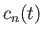, which depends entirely on the perturbation (because theare all constant if) . Note that the eigenkets, appearing in Equation (8.6), are time-independent (they are actually the eigenkets of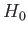evaluated at the time).

Schrödinger's time evolution equation yields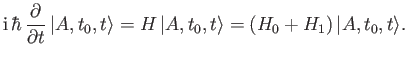(8.7)

(See Section 3.2.) It follows from Equation (8.6) that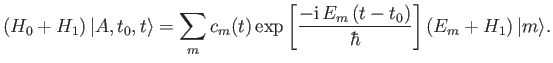(8.8)

We also have(8.9)

where use has been made of the time-independence of the kets. According to Equation (8.7), we can equate the right-hand sides of the previous two equations to obtainLeft-multiplication byyields(8.10)

where(8.11)

and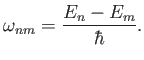(8.12)

Here, we have made use of the standard orthonormality result,. Suppose that there are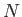linearly independent eigenkets of the unperturbed Hamiltonian. According to Equation (8.11), the time variation of the coefficients, which specify the probability of finding the system in stateat time, is determined bycoupled first-order differential equations. Note that the set of equations specified in Equation (8.11) is exact--we have made no approximations at this stage. Unfortunately, we cannot generally find exact solutions to this set, so we have to obtain approximate solutions via suitable expansions in small quantities. However, for the particularly simple case of a two-state system (i.e.,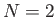), it is actually possible to solve Equation (8.11) without approximation. This solution is of great practical importance.Next: Two-State System Up: Time-Dependent Perturbation Theory Previous: Introduction
Richard Fitzpatrick 2016-01-22